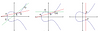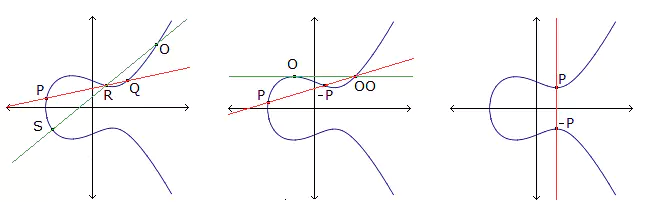# New bounds for linear codes of covering radii 2 and 3

### Journal article

D. Bartoli, A. Davydov, M. Giulietti, S. Marcugini, F. Pambianco
Cryptography and Communications, 2018

Semantic Scholar DBLP DOI
Cite

### Cite

APA
Bartoli, D., Davydov, A., Giulietti, M., Marcugini, S., & Pambianco, F. (2018). New bounds for linear codes of covering radii 2 and 3. Cryptography and Communications.

Chicago/Turabian
Bartoli, D., A. Davydov, M. Giulietti, S. Marcugini, and F. Pambianco. “New Bounds for Linear Codes of Covering Radii 2 and 3.” Cryptography and Communications (2018).

MLA
Bartoli, D., et al. “New Bounds for Linear Codes of Covering Radii 2 and 3.” Cryptography and Communications, 2018.

## Abstract

AbstractThe length function ℓq(r, R) is the smallest length of a q-ary linear code of covering radius R and codimension r. In this work we obtain new upper bounds on ℓq(2t + 1,2), ℓq(3t + 1,3), ℓq(3t + 2,3), t ≥ 1. In particular, we prove that ℓq(3,2)≤q(3lnq+lnlnq)+q3lnq+3for all q,$$\ell_{q}(3,2)\le\sqrt{q(3\ln q+\ln\ln q)}+\sqrt{\frac{q}{3\ln q}}+ 3~\text{ for all } q,$$ℓq(3,2)≤1.053qlnq$\ell _{q}(3,2)\le 1.05\sqrt {3q\ln q}$ for q ≤ 321007, ℓq(4,3)<2.8qlnq3$\ell _{q}(4,3)<2.8\sqrt {q\ln q}$ for q ≤ 6229, and ℓq(5,3)<3q2lnq3$\ell _{q}(5,3)<3\sqrt {q^{2}\ln q}$ for q ≤ 761. The new bounds on ℓq(2t + 1,2), ℓq(3t + 1,3), ℓq(3t + 2,3), t > 1, are then obtained by lift-constructions. For q a non-square the new bound on ℓq(2t + 1,2) improves the previously known ones. For many values of q≠(q′)3 and r ≠ 3t we provide infinite families of [n, n − r]q3 codes showing that ℓq(r,3)≈clnq3⋅q(r−3)/3$\ell _{q}(r,3)\thickapprox c\sqrt {\ln q}\cdot q^{(r-3)/3}$, where c is a universal constant. As far as it is known to the authors, such families have not been previously described in the literature.

Share